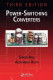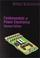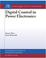Control of Power Converters

Course Description

Power converter control system structure. Static and dynamic models of power converters. Need for dynamic models. Development of dynamic models using averaging method; switching function averaging, power switch averaging. State-space models: continuous-time and discrete-time. Generalized state-space models. Linear and piecewise linear models; linearization of continuous-time and discrete-time models. Model analisys, transfer function. Control design examples; DCDC converter, renewable energy power converter system.

General Competencies

Static and dynamic power converter model development. Analisys and synthesis of power converter control systems.

Learning Outcomes

1. define power converter control system structure
2. explain static and dynamic power converter models development procedure
3. explain voltage and current mode control of power converters
4. analyze averaging and linearization of power switch and power converter
5. analyze power converters state-space models
6. apply power converter averaged and state-space models in feedback control system analisys.
7. summarize the acquired knowledge in the work with power converter laboratoy model

Forms of Teaching

Lectures

Lectures are organized through 2 teaching cycles. The first cycle consists of 7 weeks of classes and mid-term exam, a second cycle of 6 weeks of classes and final exam. Classes are conducted through a total of 15 weeks with a weekly load of 3 hours. Part of teaching is carried out interactively in the lab.

Exams

Examination consists of homeworks, mid-term exam and final exam.

Continuous Assessment Exam
Homeworks 0 % 30 % 0 % 0 %
Mid Term Exam: Written 0 % 30 % 0 %
Final Exam: Written 0 % 40 %
Exam: Written 0 % 80 %
Exam: Oral 20 %

Week by Week Schedule

1. Structure of power converter control system.
2. Static models of power converters. Overview of static models of rectifiers, inverters, DCDC converters and ACAC converters.
3. Modeling and simulation of power converters using characteristic operating intervals in transient and stationary state
4. Development of dynamic model using circuit avaraging method. Variable averaging. Passive components averaging. Averaged model of DC motor drive and up/down DCDC converter in discontinuous operating mode.
5. Averaged switching function and power switch. Generation of switching function. Averaged power converter general switching element. Example of up/down DCDC converter averaged circuit in continuous operation mode.
6. Linearization of averaged power switch. Linearization of averaged models. Example of transfer function of linearized averaged up/down DCDC converter model in continuous operation mode.
7. Application of power converter averaged models in feedback and feedforward control system analisys. Application example. Application limits of linear and nonlinear averaged models.
8. Midterm exam
9. State-space models: continuous-time and discrete-time. Features of state-space models. Example of state-space averaging for DCDC converter.
10. State-space model averaging. Generalized state-space models.
11. Power converters with voltage mode control. Power converters with current mode control.
12. Using modern software for analysis and synthesis of power converters control systems.
13. Case study: control of DCDC power converter
14. Case study: control of power converter system for renewable energy sources.
15. Final exam

Study Programmes

Control Engineering and Automation (profile)
Recommended elective courses (3. semester)
Electrical Engineering Systems and Technologies (profile)
Specialization Course (1. semester) (3. semester)

Literature

J. Kassakian, M. Schlecht, G. Verghese (2008.), Osnove energetske elektronike II. dio (prijevod), GraphisS. Ang, A. Oliva (2010.), Power Switching Converters, CRC PressR.W. Erickson (2001.), Fundamentals of Power Electronics, SpringerSimone Buso, Paolo Mattavelli (2006.), Digital Control in Power Electronics, Morgan & Claypool

General

ID 34394
Winter semester
4 ECTS
L1 English Level
L1 e-Learning
30 Lectures